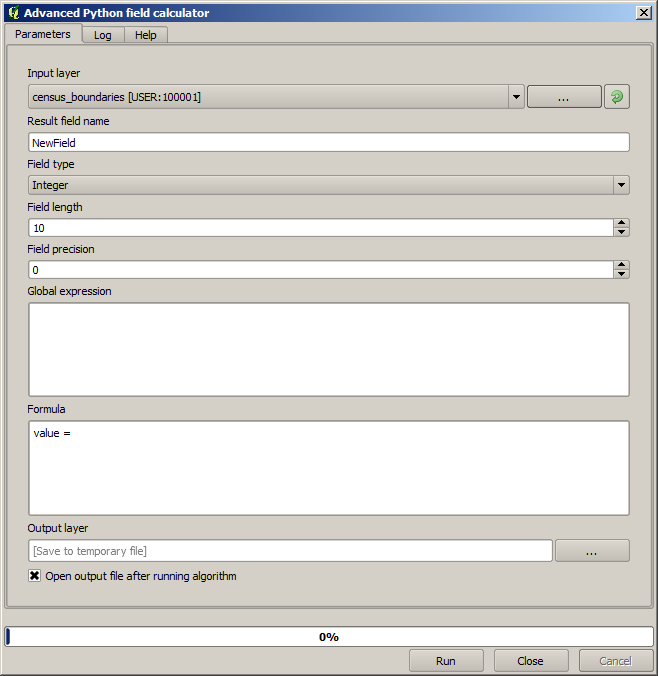# 17.11. Vector calculator¶

Note

In this lesson we will see how to add new attributes to a vector layer based on a mathematical expression, using the vector calculator.

We already know how to use the raster calculator to create new raster layers using mathematical expressions. A similar algorithm is available for vector layers, and generates a new layer with the same attributes of the input layer, plus an additional one with the result of the expression entered. The algorithm is called Field calculator and has the following parameters dialog.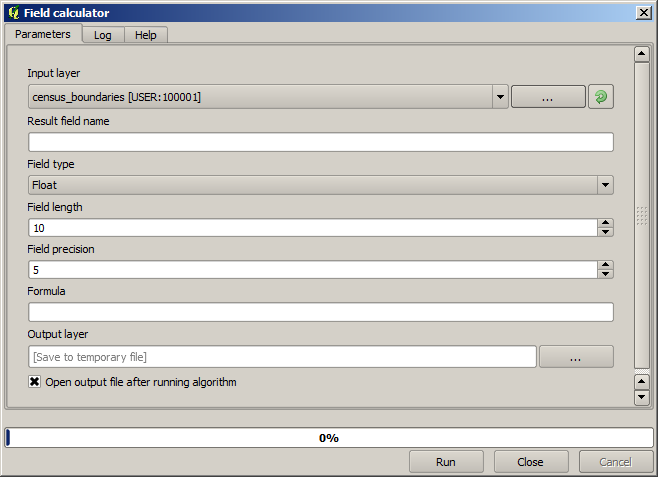Note

In newer versions of Processing the interface has changed considerably, it’s more powerful and easier to use.

Here are a few examples of using that algorithm.

First, let’s calculate the population density of white people in each polygon, which represents a census. We have two fields in the attributes table that we can use for that, namely WHITE and SHAPE_AREA. We just have to divide them and multiply by one million (to have density per square km), so we can use the following formula in the corresponding field

```( "WHITE" / "SHAPE_AREA" ) * 1000000
```

The parameters dialog should be filled as shown below.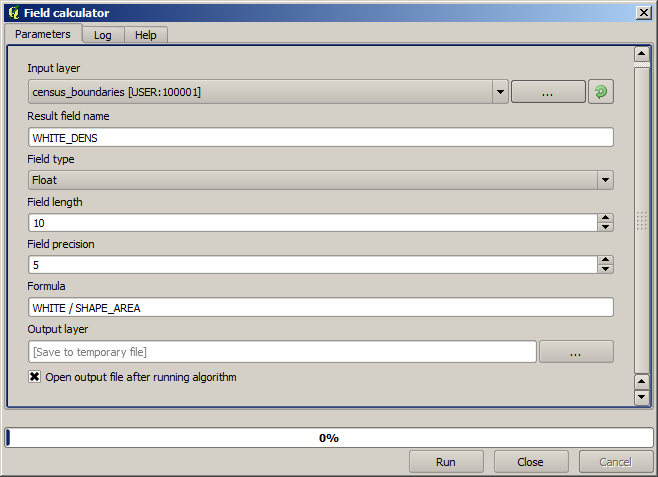This will generate a new field named WHITE_DENS

Now let’s calculate the ratio between the MALES and FEMALES fields to create a new one that indicates if male population is numerically predominant over female population.

Enter the following formula

```"MALES" / "FEMALES"
```

This time the parameters window should look like this before pressing the OK button.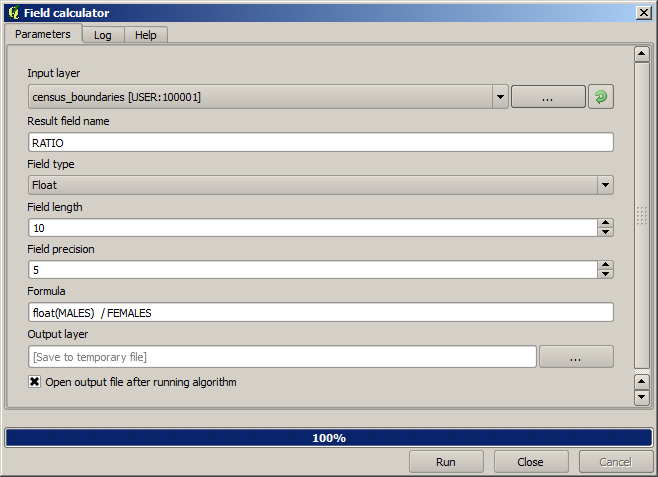In earlier version, since both fields are of type integer, the result would be truncated to an integer. In this case the formula should be: 1.0 *  "MALES"  /  "FEMALES", to indicate that we want floating point number a result.

We can use conditional functions to have a new field with male or female text strings instead of those ratio value, using the following formula:

`CASE WHEN  "MALES" > "FEMALES"  THEN 'male' ELSE 'female' END`

The parameters window should look like this.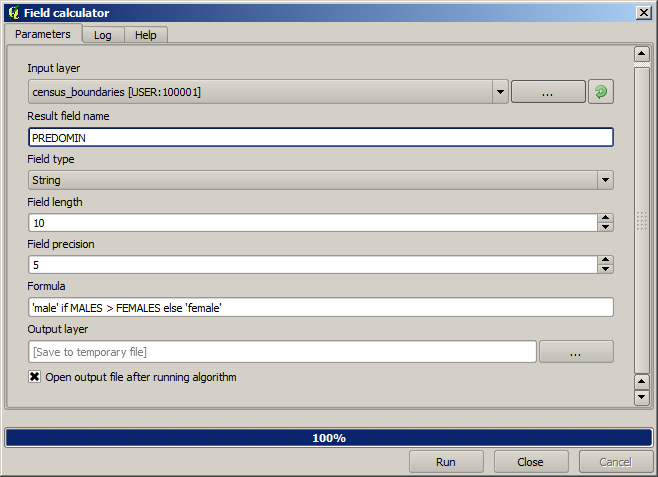A python field calculator is available in the Advanced Python field calculator, which will not be detailed here